Next: Exercises Up: Incompressible Viscous Flow Previous: Axisymmetric Stokes Flow Around

# Axisymmetric Stokes Flow In and Around a Fluid Sphere

Suppose that the solid sphere discussed in the previous section is replaced by a spherical fluid drop of radius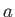. Let the drop move through the surrounding fluid at the constant velocity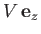. Obviously, the fluid from which the drop is composed must be immiscible with the surrounding fluid. Let us transform to a frame of reference in which the drop is stationary, and centered at the origin. Assuming that the Reynolds numbers immediately outside and inside the drop are both much less than unity, and making use of the previous analysis, the most general expressions for the stream function outside and inside the drop are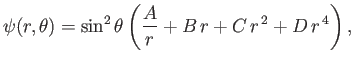(10.135)

and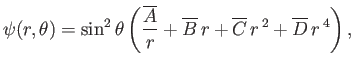(10.136)

respectively. Here,,,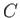, et cetera, are arbitrary constants. Likewise, the previous analysis also allows us to deduce that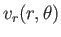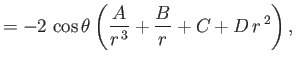(10.137)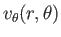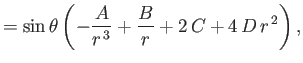(10.138)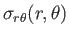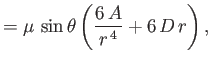(10.139)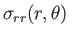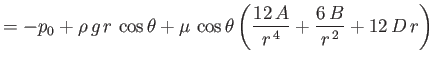(10.140)

in the region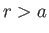, with analogous expressions in the region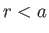. Here,,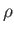,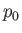are the viscosity, density, and ambient pressure of the fluid surrounding the drop. Let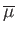,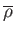, and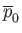be the corresponding quantities for the fluid that makes up the drop.

In the region outside the drop, the fluid velocity must asymptote to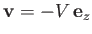at large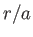. This implies that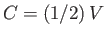and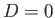. Furthermore,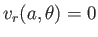--that is, the normal velocity at the drop boundary must be zero--otherwise, the drop would change shape. This constraint yields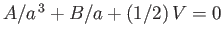.

Inside the drop, the fluid velocity must remain finite as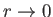. This implies that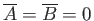. Furthermore, we again require that, which yields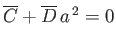.

Two additional physical constraints that must be satisfied at the interface between the two fluids are, firstly, continuity of tangential velocity--that is,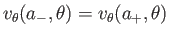--and, secondly, continuity of tangential stress--that is,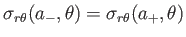. These constraints yield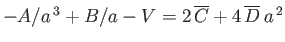and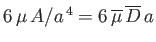, respectively.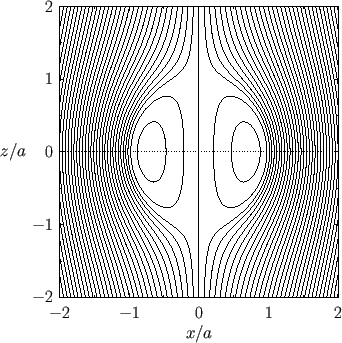At this stage, we have enough information to determine the values of,,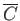, and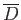. In fact, the stream functions outside and inside the drop can be shown to take the form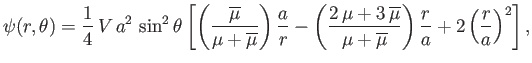(10.141)

and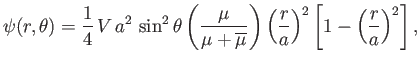(10.142)

respectively. (See Figures 10.7 and 10.8.)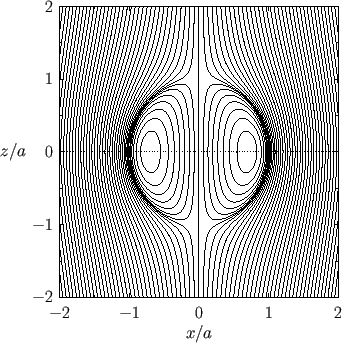The discontinuity in the radial stress across the drop boundary is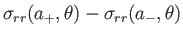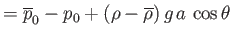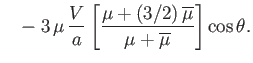(10.143)

The final physical constraint that must be satisfied at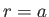is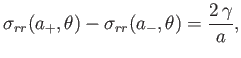(10.144)

where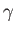is the surface tension of the interface between the two fluids. (See Section 3.3.) Hence, we obtain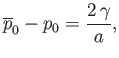(10.145)

and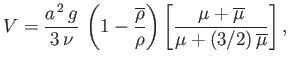(10.146)

where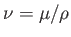is the kinematic viscosity of the surrounding fluid. The fact that we have been able to completely satisfy all of the physical constraints at the interface between the two fluids, as long as the drop moves at the constant vertical velocity, proves that our previous assumptions that the interface is spherical, and that the drop moves vertically through the surrounding fluid at a constant speed without changing shape, were correct. In the limit,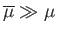, in which the drop is much more viscous than the surrounding fluid, we recover Equation (10.128): that is, the drop acts like a solid sphere. On the other hand, in the limit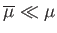, and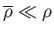, which is appropriate to an air bubble rising through a liquid, we obtain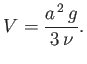(10.147)Next: Exercises Up: Incompressible Viscous Flow Previous: Axisymmetric Stokes Flow Around
Richard Fitzpatrick 2016-01-22